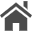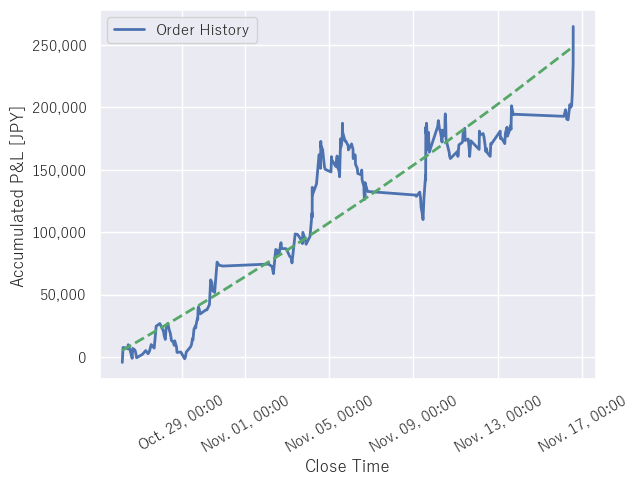Home  Updated: November 15, 2020  [日本語 / English]

# Drawing Accumulated Profit and Loss Curve in Python with seaborn

## Introduction

This page explains how to draw a profit and loss curve using Python with seaborn based on a CSV-formatted file saved by the "MQL4 Script to Save MT4's Order History to CSV file".

The CSV file is in the following format. The first line is a header (comment), and the second and subsequent lines contain the order history.

Each line is separated by commas and includes the open time, close time, number of lots, profit and loss, symbol name, and order type.

``````OpenTime,CloseTime,Lots,Profit,Symbol,Buy/Sell
2020.10.26 03:54:05,2020.10.26 04:10:03,1.41,-4371,USDJPY-cd,Sell
2020.10.26 04:21:22,2020.10.26 05:01:09,1.41,11985,USDJPY-cd,Buy
2020.10.26 07:23:36,2020.10.26 08:59:58,1.42,-426,USDJPY-cd,Buy
2020.10.26 10:00:11,2020.10.26 10:19:02,1.42,-710,USDJPY-cd,Buy
2020.10.26 10:36:15,2020.10.26 10:56:00,1.4,3360,USDJPY-cd,Sell
``````

## Program

The Python script is as follows. I use pandas to read CSV files and matplotlib with seaborn to draw the curve.

You can use the cumsum method to calculate the accumulated profit or loss.

``````import matplotlib.pyplot as plt
import matplotlib.dates as md
import seaborn as sns
import pandas as pd

# Path to the CSV file (change accordingly)
path = r'C:\HogeHoge\OrderHistory.csv'

# Reading CSV files (OpenTime and CloseTime are read as datetime types)
df = pd.read_csv(path, parse_dates=['OpenTime', 'CloseTime'])

# apply seaborn's default theme
sns.set()

# Plot x-axis as CloseTime and y-axis as accumulated profit and loss
plt.plot(df['CloseTime'], df['Profit'].cumsum(), label = 'Order History', linewidth = 2)

# Show legend
plt.legend()

# Specify the format of the x-axis scale.
ax = plt.gca()
xfmt = md.DateFormatter('%m-%d %H:%M')
ax.xaxis.set_major_formatter(xfmt)

# Rotate the x-axis scale by 30 degrees
plt.xticks(rotation = 30)

# Set the axis labels
plt.xlabel("Close Time")
plt.ylabel("Accumulated P&L [JPY]")

# Display Graph
plt.show()
``````

If you run it, you can draw a graph like the following.